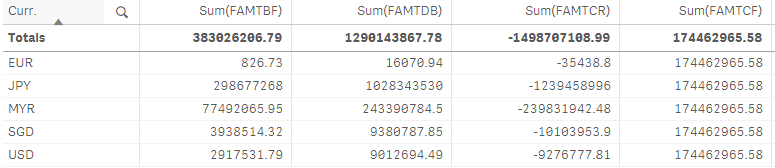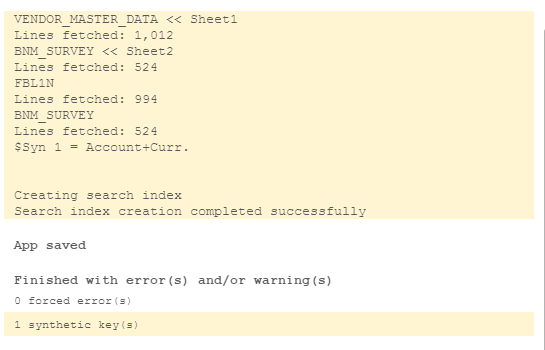# QlikView App Dev

Discussion Board for collaboration related to QlikView App Development.

Announcements
Join us at the Cloud Data and Analytics Tour! REGISTER TODAY
cancel
Showing results for
Search instead for
Did you mean:Contributor

## GROUP BY IS NOT WORKING

BNM_SURVEY:
LOAD
Account,
Curr.,
SubField(ApplyMap('VENDOR_MASTER_DATA',Account,Null()),'|',1) as [VENDOR NAME],
SubField(ApplyMap('VENDOR_MASTER_DATA',Account,Null()),'|',2) as [CTY],
"Sum of Amount in doc. curr." as [FAMTCR],
"Sum of Amount in local cur." as [LAMTCR],
"Sum of Amount in doc. curr.1" as [FAMTDB],
"Sum of Amount in local cur.1" as [LAMTDB]
FROM [lib://BNM SURVEY/FBL1N-0119-0319.xlsx]
(ooxml, embedded labels, header is 2 lines, table is Sheet2);

Left Join

LOAD
Account,
'VENDOR NAME',
CTY,
Curr.,
"Sum of FAMTBF" as [FAMTBF],
"Sum of LAMTBF" as [LAMTBF]
FROM [lib://BNM SURVEY/BF.xlsx]
(ooxml, embedded labels, table is FBL1N);

LOAD
Sum([FAMTBF])+ Sum([FAMTDB])+ Sum([FAMTCR])as [FAMTCF],
Sum([LAMTBF])+ Sum([LAMTDB])+ Sum([LAMTCR])as [LAMTCF]
Resident BNM_SURVEY

Group By
Account,
[Curr.],
[CTY];

THE RESULT OF THE SUM ON THE RESIDENT IS NOT ACHIEVE ALL THE LINE ITEM AMOUNT ARE SAME ON COLUMN SUM(FAMTCF).

CAN YOU LET ME KNOW WHERE IS THE MISTAKE.Labels (1)
• ### GROUP BY ISSUE

1 Solution

Accepted SolutionsMVP

You need to include the dimension fields in the last load. Without them, FAMTCF and LAMTCF are only global totals not associated with the dimensions in any way. Change the last load statement:

``````LOAD
Account,
[Curr.],
[CTY],
Sum([FAMTBF]) + Sum([FAMTDB]) + Sum([FAMTCR]) as [FAMTCF],
Sum([LAMTBF]) + Sum([LAMTDB]) + Sum([LAMTCR]) as [LAMTCF]
Resident BNM_SURVEY
Group By
Account,
[Curr.],
[CTY];``````

Logic will get you from a to b. Imagination will take you everywhere. - A Einstein
3 RepliesMVP

You need to include the dimension fields in the last load. Without them, FAMTCF and LAMTCF are only global totals not associated with the dimensions in any way. Change the last load statement:

``````LOAD
Account,
[Curr.],
[CTY],
Sum([FAMTBF]) + Sum([FAMTDB]) + Sum([FAMTCR]) as [FAMTCF],
Sum([LAMTBF]) + Sum([LAMTDB]) + Sum([LAMTCR]) as [LAMTCF]
Resident BNM_SURVEY
Group By
Account,
[Curr.],
[CTY];``````

Logic will get you from a to b. Imagination will take you everywhere. - A EinsteinContributor
Author

have synthetic errorMVP

The synthetic (composite) key is a result of multiple common fields. Synthetic keys are often a warning of a poorly designed data model. In this case, you could leave the synthetic key in place, or add a join instruction ahead of the last load to join the data into the fact table. Be aware that a join will add or remove records, depending on the type of join, and if the data field values are misaligned or contain duplicate values of the composite key.

Logic will get you from a to b. Imagination will take you everywhere. - A Einstein